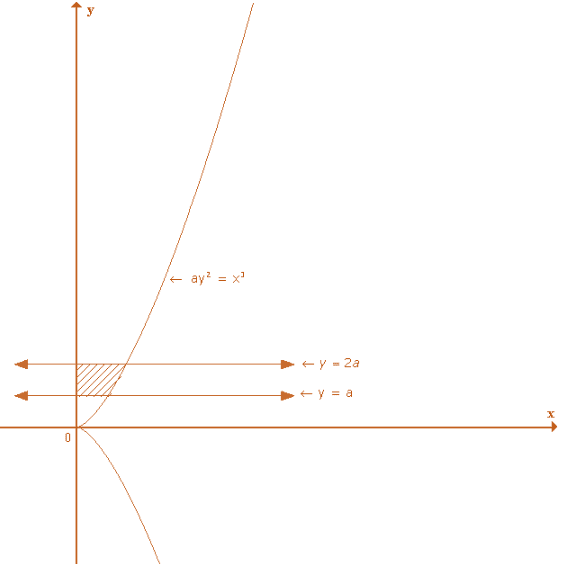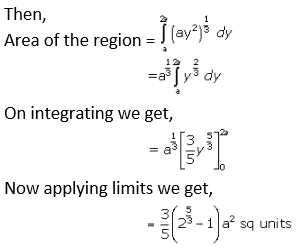# RD Sharma Solutions Class 12 Area Bounded Regions Exercise 21.2

RD Sharma Solutions for Class 12 Maths Exercise 21.2 Chapter 21 Areas of Bounded Regions are provided here. These solutions can help students clear doubts quickly and help in learning the topic effectively. This exercise deals with finding the area of the region bounded by the line and curve in the first quadrant. Students who wish to score good marks in Maths practise RD Sharma Solutions for Class 12 Maths Chapter 21.

## Download PDF of RD Sharma Solutions for Class 12 Maths Chapter 21 Exercise 2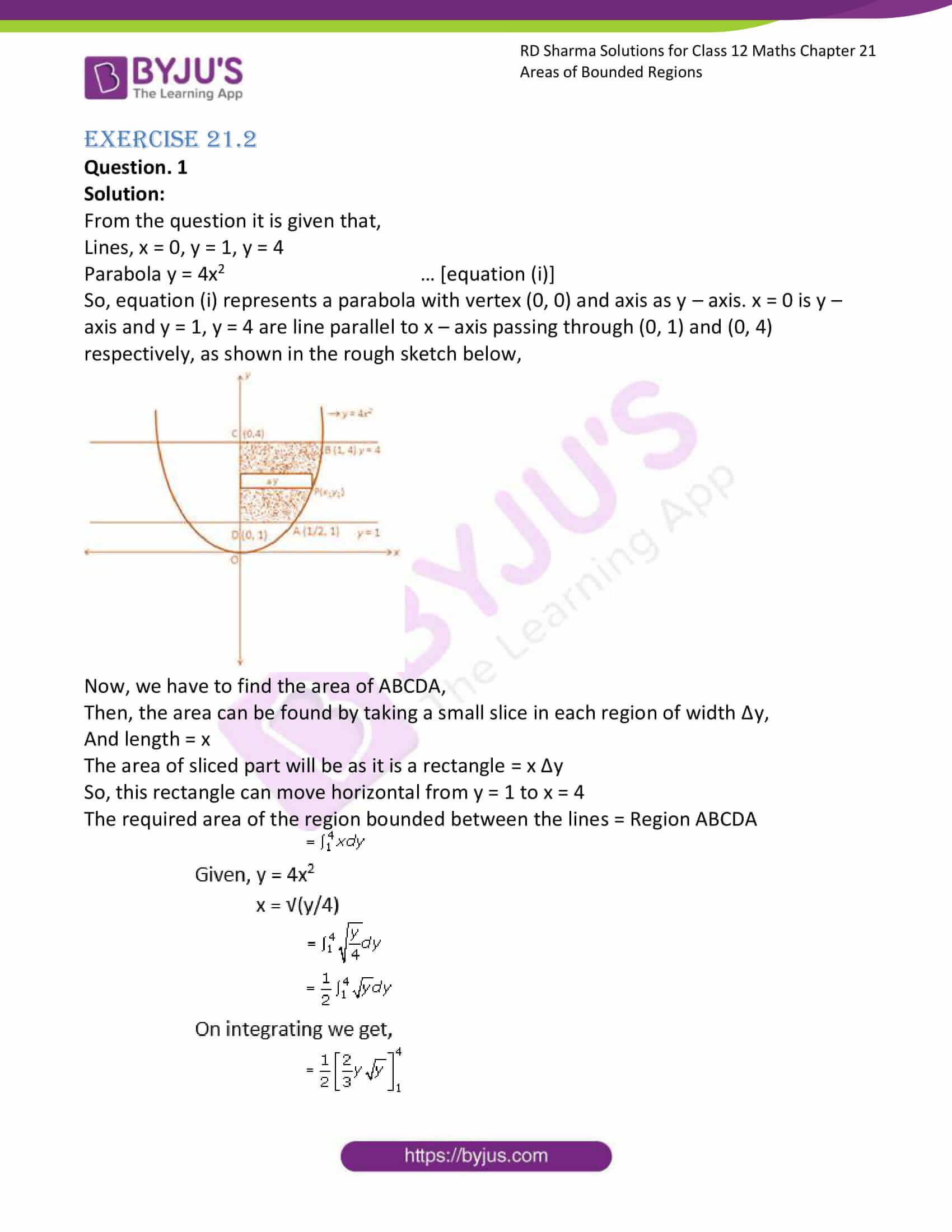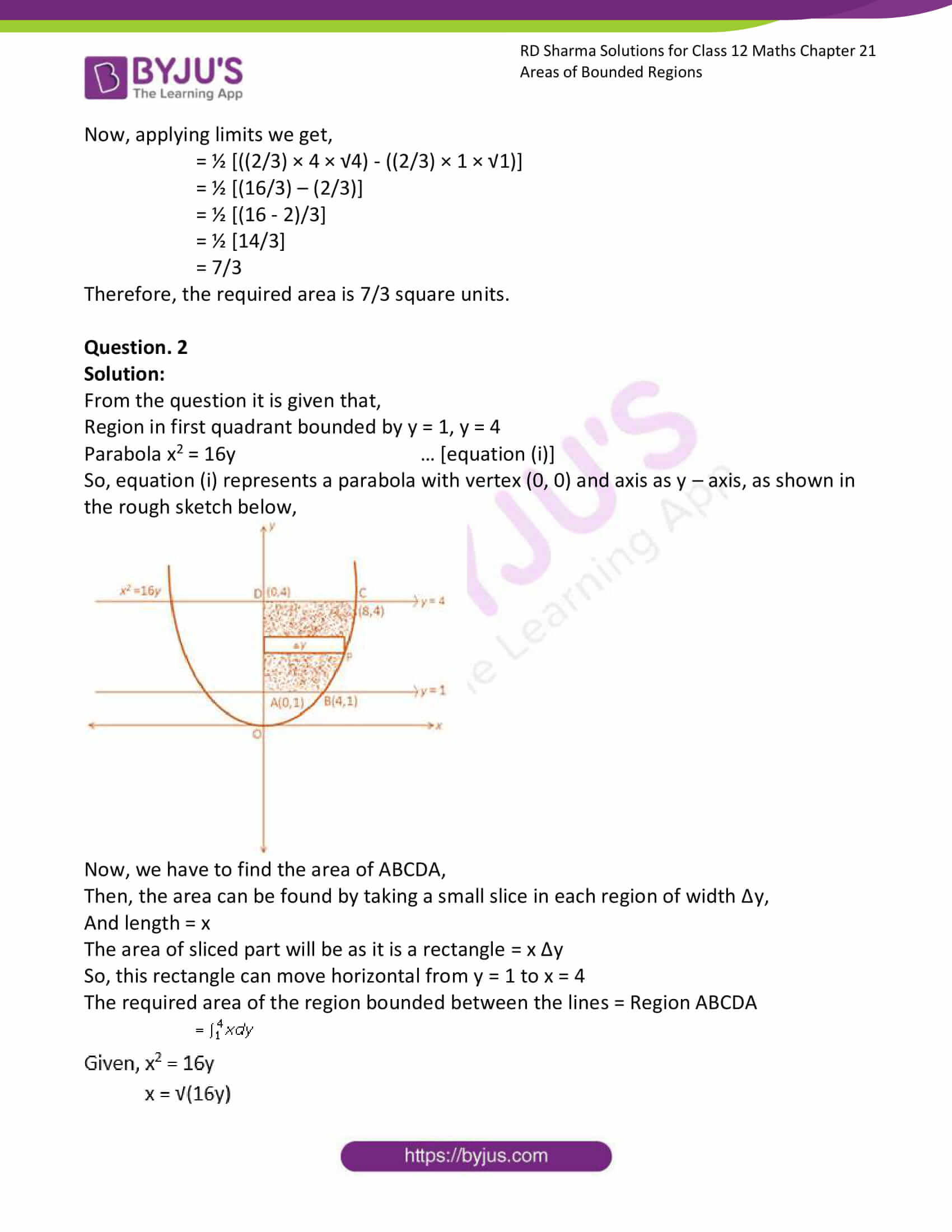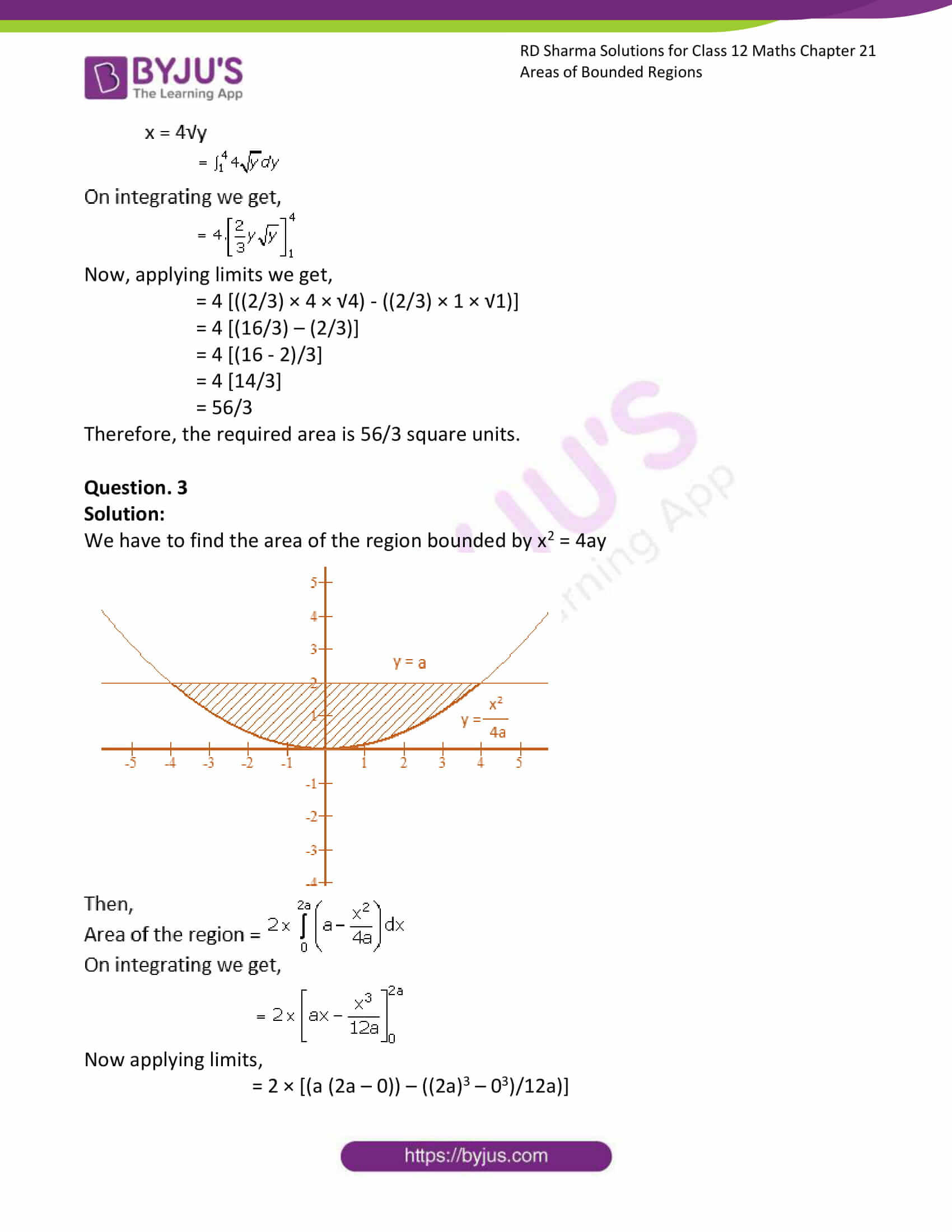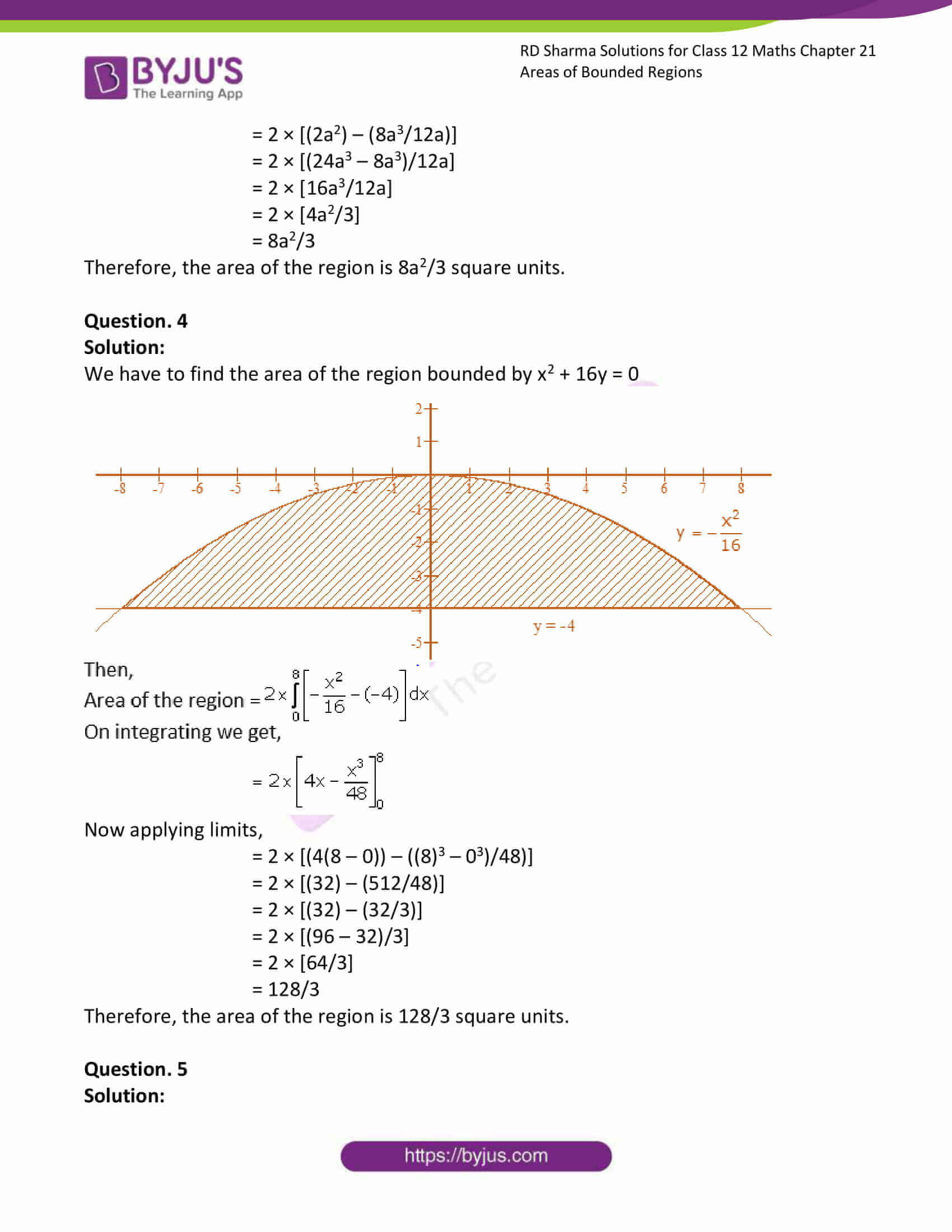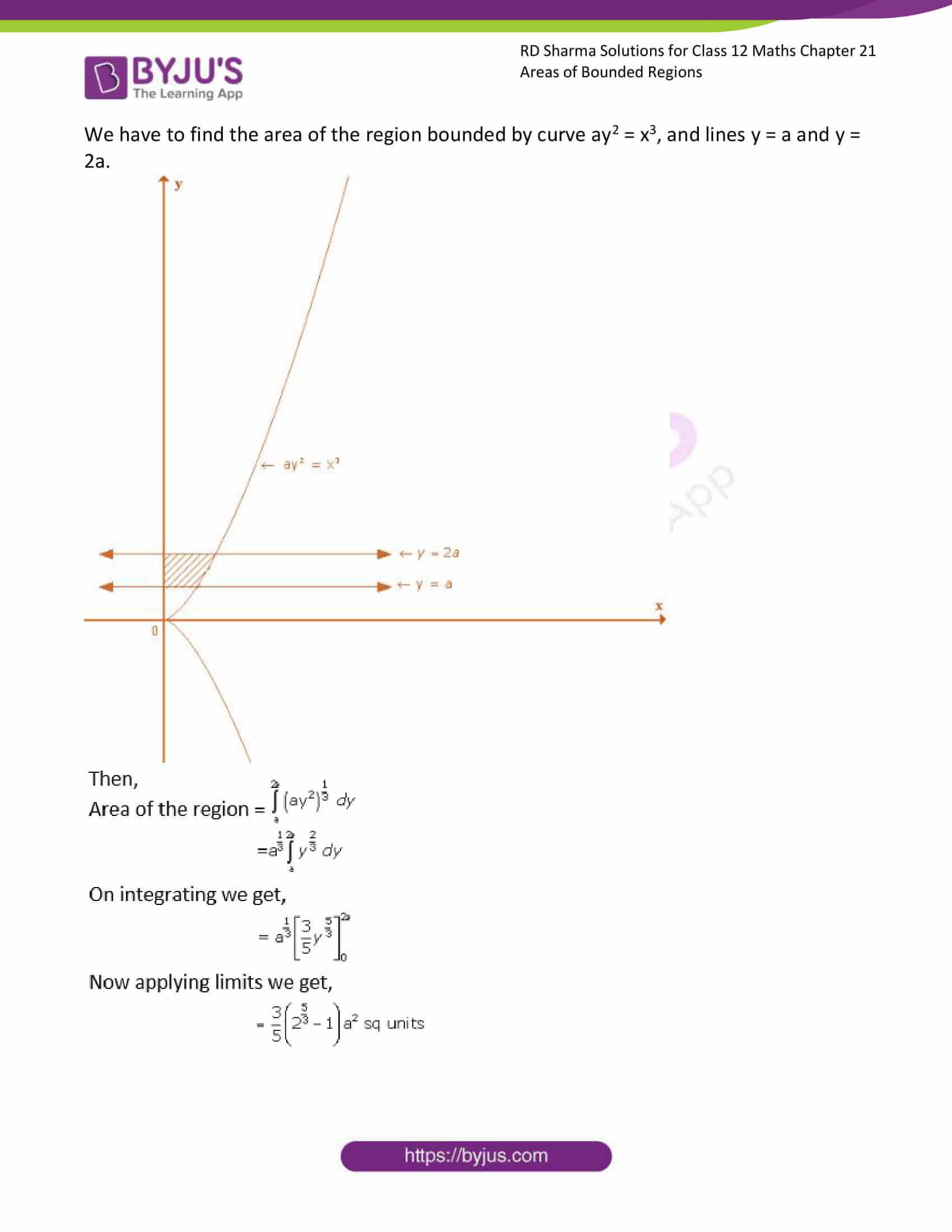### Access RD Sharma Solutions for Class 12 Maths Chapter 21 Exercise 2

EXERCISE 21.2

Question. 1

Solution:

From the question it is given that,

Lines, x = 0, y = 1, y = 4

Parabola y = 4x2 … [equation (i)]

So, equation (i) represents a parabola with vertex (0, 0) and axis as y – axis. x = 0 is y – axis and y = 1, y = 4 are line parallel to x – axis passing through (0, 1) and (0, 4) respectively, as shown in the rough sketch below,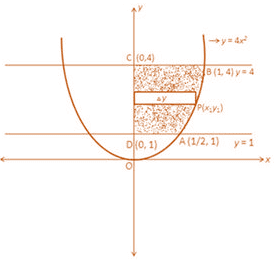Now, we have to find the area of ABCDA,

Then, the area can be found by taking a small slice in each region of width Δy,

And length = x

The area of sliced part will be as it is a rectangle = x Δy

So, this rectangle can move horizontal from y = 1 to x = 4

The required area of the region bounded between the lines = Region ABCDA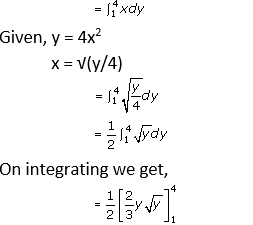Now, applying limits we get,

= ½ [((2/3) × 4 × √4) – ((2/3) × 1 × √1)]

= ½ [(16/3) – (2/3)]

= ½ [(16 – 2)/3]

= ½ [14/3]

= 7/3

Therefore, the required area is 7/3 square units.

Question. 2

Solution:

From the question it is given that,

Region in first quadrant bounded by y = 1, y = 4

Parabola x2 = 16y … [equation (i)]

So, equation (i) represents a parabola with vertex (0, 0) and axis as y – axis, as shown in the rough sketch below,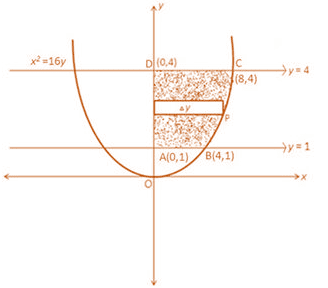Now, we have to find the area of ABCDA,

Then, the area can be found by taking a small slice in each region of width Δy,

And length = x

The area of sliced part will be as it is a rectangle = x Δy

So, this rectangle can move horizontal from y = 1 to x = 4

The required area of the region bounded between the lines = Region ABCDA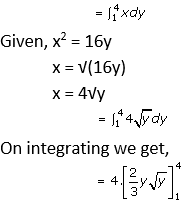Now, applying limits we get,

= 4 [((2/3) × 4 × √4) – ((2/3) × 1 × √1)]

= 4 [(16/3) – (2/3)]

= 4 [(16 – 2)/3]

= 4 [14/3]

= 56/3

Therefore, the required area is 56/3 square units.

Question. 3

Solution:

We have to find the area of the region bounded by x2 = 4ay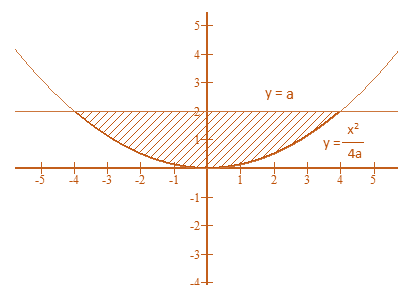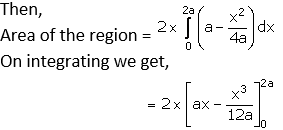Now applying limits,

= 2 × [(a (2a – 0)) – ((2a)3 – 03)/12a)]

= 2 × [(2a2) – (8a3/12a)]

= 2 × [(24a3 – 8a3)/12a]

= 2 × [16a3/12a]

= 2 × [4a2/3]

= 8a2/3

Therefore, the area of the region is 8a2/3 square units.

Question. 4

Solution:

We have to find the area of the region bounded by x2 + 16y = 0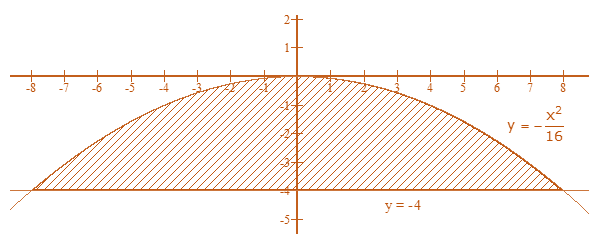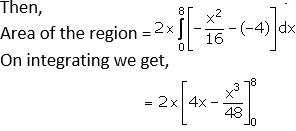Now applying limits,

= 2 × [(4(8 – 0)) – ((8)3 – 03)/48)]

= 2 × [(32) – (512/48)]

= 2 × [(32) – (32/3)]

= 2 × [(96 – 32)/3]

= 2 × [64/3]

= 128/3

Therefore, the area of the region is 128/3 square units.

Question. 5

Solution:

We have to find the area of the region bounded by curve ay2 = x3, and lines y = a and y = 2a.